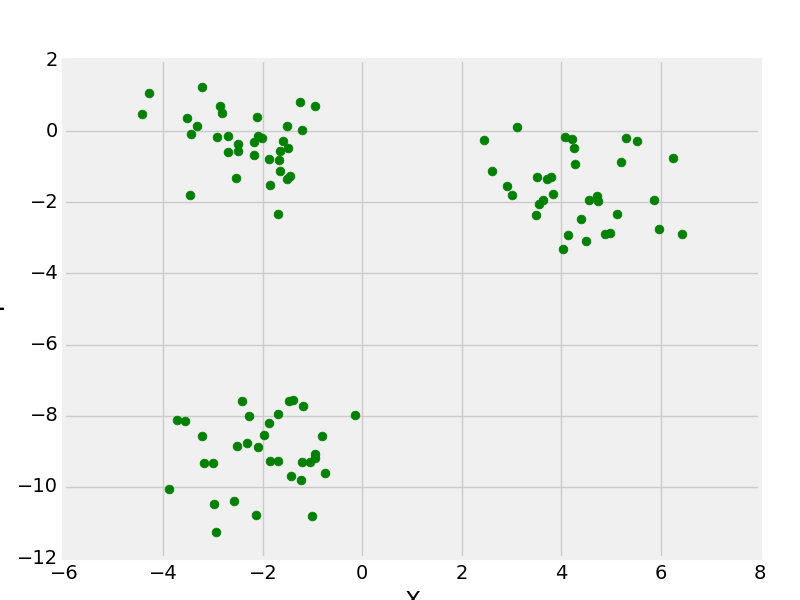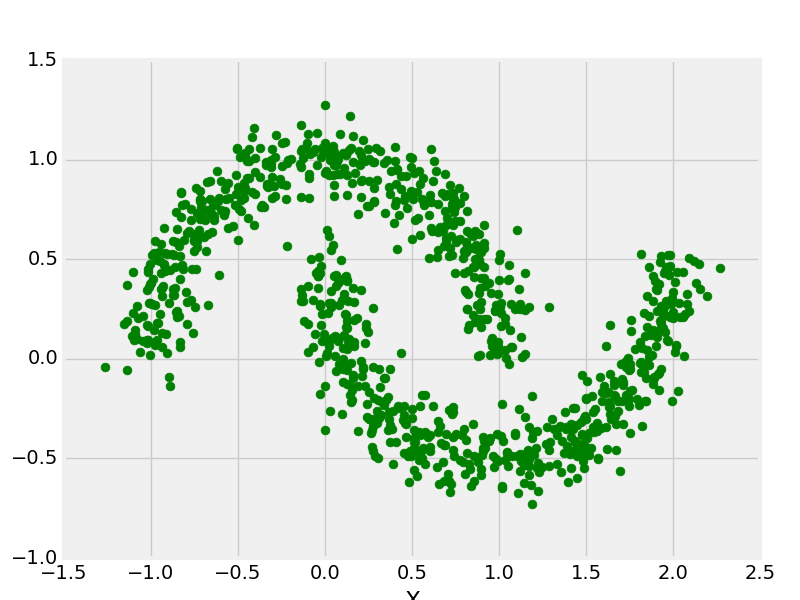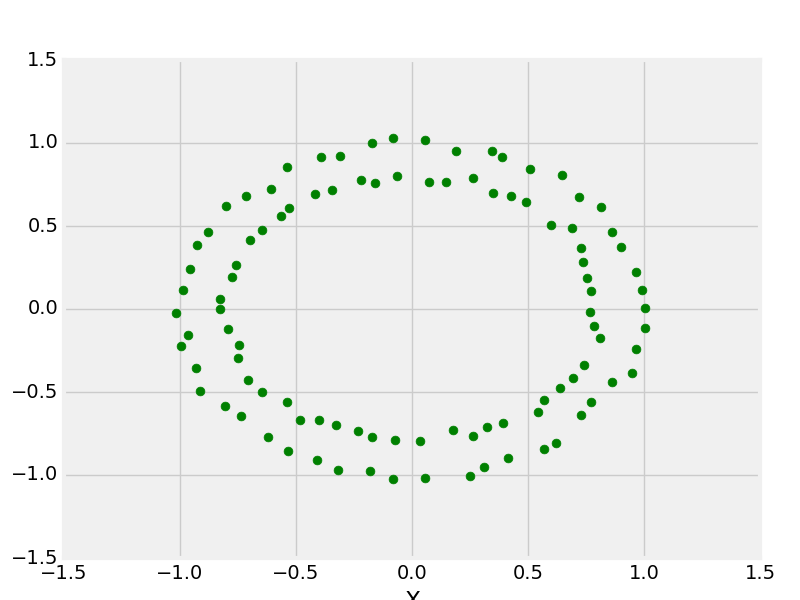# Python | Create Test DataSets using Sklearn

Python’s Sklearn library provides a great sample dataset generator which will help you to create your own custom dataset. It’s fast and very easy to use. Following are the types of samples it provides.

For all the above methods you need to import `sklearn.datasets.samples_generator`.

 `# importing libraries ` `from` `sklearn.datasets.samples_generator ` ` `  `# matplotlib for ploting ` `from` `matplotlib ``import` `pyplot as plt  ` `from` `matplotlib ``import` `style `

``` sklearn.datasets.make_blobs ```

 `# Creating Test DataSets using sklearn.datasets.make_blobs ` `from` `sklearn.datasets.samples_generator ``import` `make_blobs ` `from` `matplotlib ``import` `pyplot as plt  ` `from` `matplotlib ``import` `style ` ` `  `style.use(``"fivethirtyeight"``) ` ` `  `X, y ``=` `make_blobs(n_samples ``=` `100``, centers ``=` `3``,  ` `               ``cluster_std ``=` `1``, n_features ``=` `2``) ` ` `  `plt.scatter(X[:, ``0``], X[:, ``1``], s ``=` `40``, color ``=` `'g'``) ` `plt.xlabel(``"X"``) ` `plt.ylabel(``"Y"``) ` ` `  `plt.show() ` `plt.clf() `

Output:make_blobs with 3 centers

``` sklearn.datasets.make_moon ```

 `# Creating Test DataSets using sklearn.datasets.make_moon ` `from` `sklearn.datasets.samples_generator ``import` `make_moon ` `from` `matplotlib ``import` `pyplot as plt  ` `from` `matplotlib ``import` `style ` ` `  `X, y ``=` `make_moons(n_samples ``=` `1000``, noise ``=` `0.1``) ` `plt.scatter(X[:, ``0``], X[:, ``1``], s ``=` `40``, color ``=``'g'``) ` `plt.xlabel(``"X"``) ` `plt.ylabel(``"Y"``) ` ` `  `plt.show() ` `plt.clf() `

Output:make_moons with 1000 data points

``` sklearn.datasets.make_circle ```

 `# Creating Test DataSets using sklearn.datasets.make_circles ` `from` `sklearn.datasets.samples_generator ``import` `make_circles ` `from` `matplotlib ``import` `pyplot as plt  ` `from` `matplotlib ``import` `style ` ` `  `style.use(``"fivethirtyeight"``) ` ` `  `X, y ``=` `make_circles(n_samples ``=` `100``, noise ``=` `0.02``) ` `plt.scatter(X[:, ``0``], X[:, ``1``], s ``=` `40``, color ``=``'g'``) ` `plt.xlabel(``"X"``) ` `plt.ylabel(``"Y"``) ` ` `  `plt.show() ` `plt.clf() `

Output:make _circle with 100 data points

My Personal Notes arrow_drop_upCheck out this Author's contributed articles.

If you like GeeksforGeeks and would like to contribute, you can also write an article using contribute.geeksforgeeks.org or mail your article to contribute@geeksforgeeks.org. See your article appearing on the GeeksforGeeks main page and help other Geeks.

Please Improve this article if you find anything incorrect by clicking on the "Improve Article" button below.

Article Tags :
Practice Tags :

Be the First to upvote.

Please write to us at contribute@geeksforgeeks.org to report any issue with the above content.